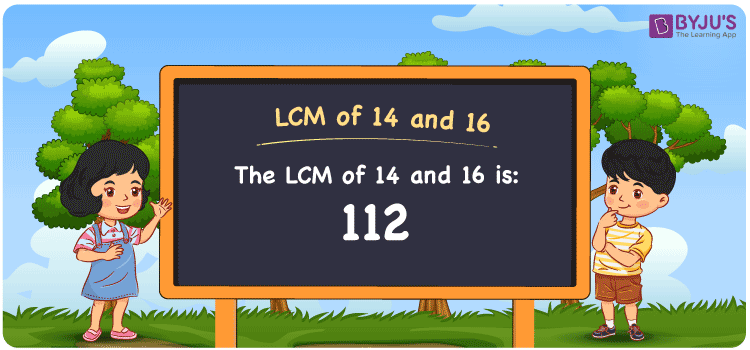Checkout JEE MAINS 2022 Question Paper Analysis : Checkout JEE MAINS 2022 Question Paper Analysis :

# LCM of 14 and 16

LCM of 14 and 16 is 112. Get to know how the least common multiple of 14 and 16 is obtained by taking the common multiples into consideration. The multiples of 14 are (14, 28, 42, 56, ….) and the multiples of 16 are (16, 32, 48, 64, 80,….). You can find the LCM of two numbers using the prime factorisation method, by listing out the multiples and division methods.

## What is LCM of 14 and 16?

The answer to this question is 112. The LCM of 14 and 16 using various methods is shown in this article for your reference. The LCM of two non-zero integers, 14 and 16, is the smallest positive integer 112 which is divisible by both 14 and 16 with no remainder.## How to Find LCM of 14 and 16?

LCM of 14 and 16 can be found using three methods:

• Prime Factorisation
• Division method
• Listing the multiples

### LCM of 14 and 16 Using Prime Factorisation Method

The prime factorisation of 14 and 16, respectively, is given by:

14 = 2 x 7 = 2¹ x 7¹

16 = 2 x 2 x 2 x 2 = 2

LCM (14, 16) = 112

### LCM of 14 and 16 Using Division Method

We’ll divide the numbers (14, 16) by their prime factors to get the LCM of 14 and 16 using the division method (preferably common). The LCM of 14 and 16 is calculated by multiplying these divisors.

 2 14 16 2 7 8 2 7 4 2 7 2 7 7 1 x 1 1

No further division can be done.

Hence, LCM (14, 16) = 112

### LCM of 14 and 16 Using Listing the Multiples

To calculate the LCM of 14 and 16 by listing out the common multiples, list the multiples as shown below

 Multiples of 14 Multiples of 16 14 16 28 72 42 48 56 64 70 80 84 96 98 112 112 128 126 144

The smallest common multiple of 14 and 16 is 112.

Therefore LCM (14, 16) = 112

## Video Lesson on Applications of LCM## LCM of 14 and 16 Solved Examples

Question: If the product of two numbers is 224 and the GCD is 2, determine the LCM.

Solution:

It is given that

Product of two numbers = 224

GCD = 2

We know that

LCM x GCD = Product of two numbers

LCM = Product/GCD

LCM = 224/2

LCM = 112

Hence, the LCM is 112.

## Frequently Asked Questions on LCM of 14 and 16

### What is the LCM of 14 and 16?

The LCM of 14 and 16 is 112. To determine the LCM, we have to find the multiples of 14 and 16 first and the smallest multiple exactly divisible by 14 and 16 should be found.

### Write the methods used to get the LCM of 14 and 16.

The methods used to get the LCM of 14 and 16 are the Division Method, Prime Factorization Method and Listing multiples.

### Determine the GCF if the LCM of 14 and 16 is 112.

LCM x GCF = 14 x 16

Given

LCM of 14 and 16 = 112

112 x GCF = 224

GCF = 224/112 = 2